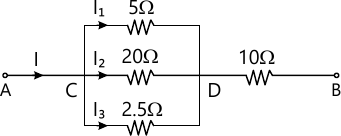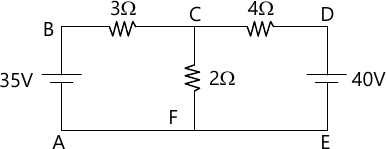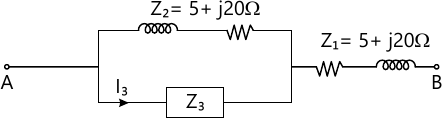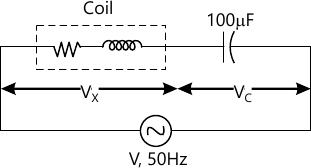MORE IN Basic Electrical Engg.
VTU First Year Engineering (P Cycle) (Semester 1)
Basic Electrical Engg.
December 2015
Total marks: --
Total time: --
INSTRUCTIONS
(1) Assume appropriate data and state your reasons
(2) Marks are given to the right of every question
(3) Draw neat diagrams wherever necessary

1 (a) Compare electric circuit and magnetic circuit.
5 M
1 (b) For the circuit shown in Fig Q1(b), the total power dissipated is 488W. Calculate the current flowing in each resistance and pd between A and B.5 M
1 (c) Derive an expression for the energy stored in the magnetic field.
5 M
1 (d) A coil of 200 turns of wire is wound on a magnetic circuit of reluctance 2000 AT/wb. If a circuit of 1A flowing in the coil is reversed in 10 sec, find the average emf induced in coil.
5 M

2 (a) Define the following:
i) Statically induced emf
ii) Dynamically induced emf
iii) Go-efficient of coupling. Give example for (i) and (ii) and expression for (iii).
6 M
2 (b) A conductor of length 0.5m situated in and at right angles to a uniform magnetic field of flux density 1 Wb/m2 moves with a velocity of 40 m/s. Calculate the emf induced in the conductor. What will be the emf induced if the conductor moves at an angle 60° to the field.
4 M
2 (c) State and explain Kirchoff's laws.
4 M
2 (d) For the Fig Q2(d) calculate the current in 2Ω resistor.6 M

3 (a) With a neat diagram showing important parts of DC machine and explain important features of the parts shown.
8 M
3 (b) A 230V DC shunt motor takes a no load current of 3A and runs at 1100 rpm. If the full load current is 41A. Find the speed on full load. Assume armature resistance 0.25Ω and shunt filed resistance 230Ω.
6 M
3 (c) With neat sketch, explain the working of dynamometer type wattmeter.
6 M

4 (a) With a neat diagram, explain the principle of operation of single phase induction type energy meter.
6 M
4 (b) Derive the emf equation of a DC generator.
6 M
4 (c) A 440 V Dc shunt motor takes an armature current of 20 A and runs at 500 rpm. The armature resistance is 0.6 Ω. If the flux is reduced by 30% and the torque is increased by 40% calculate the new value of armature current and speed.
8 M

5 (a) Define the following with reference to AC quantities: i) Instantaneous value
ii) Frequency
iii) Time period
iv) Form factor
v) Peak factor.
5 M
5 (b) When 220 V AC supply is applied across AB terminals for the circuit shown in Fig. 5(b), the input is 3.25 KW and the current is 20A. Find the current through Z3.9 M
5 (c) Explain the working of three-way control of lamp with the help of switching table.
6 M

6 (a) With a neat diagram explain the working of RCCB.
6 M
6 (b) Prove that a pure capacitor do not consume any power.
6 M
6 (c) A coil of p.f. 0.6 is in series with a 100 μF capacitor. When connected to a 50Hz supply the p.d. across the coil is the p.d. across the capacitor. Find the resistance and inductance of the coil for the circuit shown in Fig. Q6(c)8 M

7 (a) List the advantages of 3-ph system over 1-ph system.
6 M
7 (b) Three 50Ω resistor are connected in star across 400V 3-ph supply:
i) Find phase current, line current and power drawn from supply
ii) What would be the above values if one of the resistors were disconnected?
6 M
7 (c) What are the advantages of rotating field type alternator?
3 M
7 (d) A 2-pole, 3-ph alternator running at 3000 rpm has 42 armature slots with 2 conductors in each slot. Calculate the flux/pole required to generate a phase voltage of 1100 V. Assume kd=0.97, and full pitch winding.
5 M

8 (a) Derive an emf equation of alternator.
6 M
8 (b) A 12 pole 500 rpm star connected alternator has 48 slots with 15 conductors/slot the flux/pole is 0.02 Wb and is distributed sinusoidally. The winding factor is 0.97 calculate the line emf.
4 M
8 (c) Derive a relation between line current and phase current in case of 3-ph Delta connected load.
6 M
8 (d) Three similar coils are connected in delta across a 3-ph supply. The two wattmeters connected to measure the input power indicate 12 KW and 7 KW. Calculate:
i) Power input
ii) Power factor of the load.
4 M

9 (a) Explain various losses in transformer. How these losses can be minimized?
5 M
9 (b) A 600 KVA transformer has an efficiency of 92% both at full load unity p.f. and half load 0.9 p.f. Determine its efficiency at 75% of full load and 0.9 p.f.
7 M
9 (c) List the differences between squirrel cage and wound rotor induction motor.
4 M
9 (d) A 4-pole, 3-ph 1M is supplied from 50Hz supply. Find its synchronous speed. On full load its speed observed to be 1410 rpm. Calculate its full load slip.
4 M

10 (a) Explain the necessity of starters in 3-ph induction motor.
4 M
10 (b) A 3-ph 1M with 4-pole is supplied from an alternator having 6-poles and running at 1000 rpm. Calculate:
i) The synchronous speed of 1M
ii) Its speed when slip is 0.04
iii) Frequency of the rotor emf when the speed is 600 rpm.
6 M
10 (c) Define the voltage regulation of a transformer. What is its importance?
4 M
10 (d) A 500 KVA transformer has N1: N2=300:20. The primary winding is connected to a 2200 V,50 Hz supply calculate:
i) Secondary voltage on no load.
ii) Approximate values of primary and secondary currents on full load.
iii) The maximum value of the flux.
6 M

More question papers from Basic Electrical Engg.